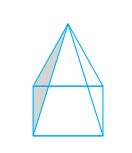Q

# A square pyramid has a square as its base.

A square pyramid has a square as its base.

Faces : _______

Edges : _______

Corners : _______Views

In a square pyramid, the number of

Faces =           (Four triangular faces and one square face)

Edges =           (Four edges of the square base and other four joining at the top)

Corners =

Exams
Articles
Questions